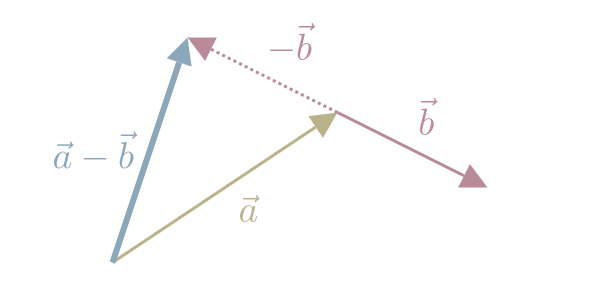## Vector SubtractionWe subtract one Vector from another by subtracting the corresponding components.

$\vec{a} - \vec{b} = \begin{bmatrix} a_1 \\ a_2 \end{bmatrix} - \begin{bmatrix} b_1 \\ b_2 \end{bmatrix} = \begin{bmatrix} a_1 - b_1 \\ a_2 - b_2 \end{bmatrix}$

Another way to think of vector subtraction, is Vector Addition with the negative of a vector: $\vec{a} - \vec{b} = \vec{a} + (-\vec{b})$

We can visualize Vector subtraction as follows:

1. draw the 1st Vector
2. draw the 2nd negative Vector as a vector pointing in the opposite direction
3. Draw a line from the tail of the 1st Vector to the tip of the negative 2nd Vector.Like Vector Addition, we can only subtract two vectors with the same number of dimensions.

3Blue1Brown (2016)

#### References

3Blue1Brown. Vectors | Chapter 1, Essence of linear algebra. August 2016. URL: https://www.youtube.com/watch?v=fNk_zzaMoSs.